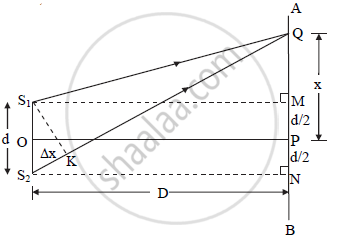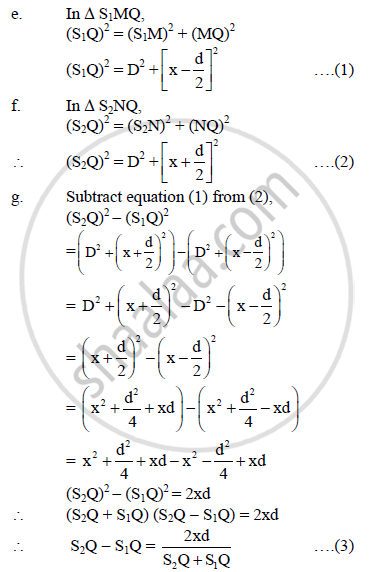HSC Science (Electronics) 12th Board ExamMaharashtra State Board
Share

# Using Analytical Method for Interference Bands, Obtain an Expression for Path Difference Between Two Light Waves. - HSC Science (Electronics) 12th Board Exam - Physics

#### Question

Using analytical method for interference bands, obtain an expression for path difference between two light waves.

#### Solution

a) Let S1 and S2 be the two coherent monochromatic sources which are separated by short distance d. They emit light waves of wavelength λ

b) Let D = horizontal distance between screen and source

c) Draw S1M and S2N ⊥ AB

OP = perpendicular bisector of slit.

Since S1P = S2P, the path difference between waves reaching P from S1 and S2 is zero, therefore there is a bright point at P.

d) Consider a point Q on the screen which is at a distance x from the central point P on the screen. Light waves from S1 and S2 reach at Q simultaneously by covering path S1Q and S2Q, where they superimposeh).  If x << D and d << D then,

S1Q ≈ S2Q ≈ D

S2Q + S1Q = 2D

∴ Equation (3) becomes,

S_2Q - S_1Q = (2xd)/(2D)

:. S_2Q - S_1Q = (xd)/D

:.trianglex = (xd)/D                .........4

Equation (4) gives the path difference of two interfering light waves

Is there an error in this question or solution?

#### APPEARS IN

2015-2016 (July) (with solutions)
Question 7.1 | 3.00 marks

#### Video TutorialsVIEW ALL 

Solution Using Analytical Method for Interference Bands, Obtain an Expression for Path Difference Between Two Light Waves. Concept: Wave Theory of Light.
S